Search IntMath
Close

450+ Math Lessons written by Math Professors and Teachers

5 Million+ Students Helped Each Year

1200+ Articles Written by Math Educators and Enthusiasts

Simplifying and Teaching Math for Over 23 Years

# Quadratic formula by completing the square - easier method

By Murray Bourne, 14 Feb 2011

Most math text books derive the Quadratic Formula as follows:

To find the roots of a quadratic equation in the form ax2 + bx + c = 0, follow these steps:

(i) If a does not equal 1, divide each side by a (so that the coefficient of the x2 is 1).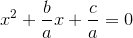(ii) Rewrite the equation with the constant term on the right side.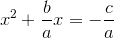(iii) Complete the square by adding the square of one-half of the coefficient of x (this is the square of b/2a) to both sides.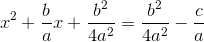(iv) Write the left side as a perfect square and simplify the right side.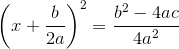(v) Equate and solve.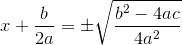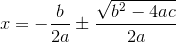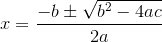Now, that's pretty messy since there is a lot going on.

## Alternative Derivation of Quadratic Formula

Here's a simpler process.
Once again, we start with an equation in the form (and call it Equation ):Multiply both sides by 4a: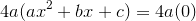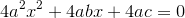Now, go back to the starting equation , find the coefficient of x (it's b) and square it (we get b2). Add that number to both sides of our equation: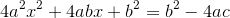Write the left side as a perfect square: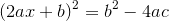Solve for x: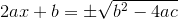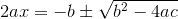I hope you find that easier to follow than the more common method (presented at top).

## Solving a quadratic equation using the alternative method of completing the square

Question: Solve the quadratic equation using completing the square: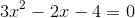Answer: In this example. a = 3, so 4a = 12. We multiply both sides by 12: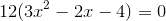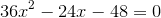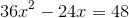Now, in the question, b = −2. We square this (b2 = 4) and add it to both sides: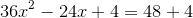Next, write the left side as a perfect square: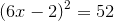Solve for x: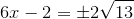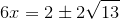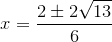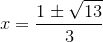What are your thoughts on this method? Is it easier for you?

[Hat tip to reader Lemmie, who sent me this method.]

### 14 Comments on “Quadratic formula by completing the square - easier method”

1. pat ballew says:

This method was made popular around 1815 as the "Hindoo" method by Edward Strachey's translation of the Bija Ganita by Bhaskara. It was known as early as the ninth century and called the "pulverizer".

Some more complete history can be found in an article I wrote called Twenty Ways to Solve a Quadratic.. Section six [no longer available]

2. Murray says:

Thanks for the extra background, Pat!

3. Sue VanHattum says:

Thanks, Pat!

And James Tanton has 2 great videos which explain this method, at https://www.youtube.com/watch?v=OZNHYZXbLY8 and https://www.youtube.com/watch?v=bjH1HphOZ1Y.

4. Murray says:

Thanks for the video links, Sue.

5. elayarajah says:

excellent, but as I am mathematician, is there any theory related to the alternative ways?

6. Hope says:

The alternative seems pretty straightforward and simple. What is the rationale behind multiplying by 4a?

7. Murray says:

@elayarajah0 and Hope: The "multiply by 4a" step is there to give us a perfect square (4a2x2) in the first term.

Similarly, the "+ b2" is there to give us a perfect square in the 3rd term.

The result - all of the left hand side is a perfect square and can be easily factored.

8. Jemal Kemal Nigo says:

I like the alternative method to solve the quadiratic equation. It makes to see that there exists different methods to solve quadratic equations. for its rationale, one has to search. we have not only to ask for every thing. We have also to add some thing to the problem as our own contribution. By the way I am also always try to find an alternative methods for solving different Mathematical entities and even try to formulate different mathematical rules. I may join you if possible
thank you.

9. Murray says:

@Jemal: It's great that you try to solve things using alternative methods. This is a good way to really understand something - and to advance mathematics.

10. Omari Ngondo says:

Thanks Murray

11. Chad Walls - Math Tutor says:

I like the first method best. As a high school math tutor I have completed the square and used the quadratic formula to solve for the zeros numerous times but have actually never had to derive the quadratic formula. I find this actually quite interesting and think I could use it as I tutor to help students bother remember how to complete the squares and memorize the quadratic formula. Thanks!

12. nicy12 says:

it really helps me a lot considering that it is very hard to understand the first process or derivation. now, i understand how it works. thanks!

13. Thompson Jumoke says:

The quadratic equation derivation makes the completing the square look more easier than i expectedd.Actually, i prefer the first method,it is more understandable.

14. MORRIS M. MAKAU says:

Every ticking second is a learning opportunity.Thanks, I have acquired a new layer of knowledge on quadratic equation solving technique that I can deploy to my students later in my teaching career after am done with my studies.It is imperative that teachers stay a head of the pack in discovery and acquisition of new knowledge. This helps to build a strong foundation on confidence among your students.

### Comment Preview

HTML: You can use simple tags like <b>, <a href="...">, etc.

To enter math, you can can either:

1. Use simple calculator-like input in the following format (surround your math in backticks, or qq on tablet or phone):
a^2 = sqrt(b^2 + c^2)
(See more on ASCIIMath syntax); or
2. Use simple LaTeX in the following format. Surround your math with $$ and $$.
$$\int g dx = \sqrt{\frac{a}{b}}$$
(This is standard simple LaTeX.)

NOTE: You can mix both types of math entry in your comment.